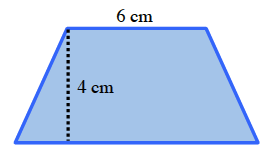### Home > CCAA > Chapter 8 Unit 7 > Lesson CC2: 8.2.2 > Problem8-47

8-47.

Draw an isosceles trapezoid (a trapezoid with two equal, non-parallel sides). Label the top base $6$ cm and the height $4$ cm. If the area of this trapezoid is $36$ square cm, what is the length of the other base? You may find it helpful to use the 5-D Process to write an equation to solve.Note: Figure not necessarily drawn to scale.

Try different values to solve for the other base.

$\text{Area}=\frac{1}{2}\cdot(b_1+b_2)\cdot h$

$12$ cm. Be sure to follow the Order of Operations.